• +91 9971497814
• info@interviewmaterial.com

# RD Chapter 1- Real Numbers Ex-1.5 Interview Questions Answers

### Related Subjects

Question 1 :
Show that the following numbers are irrational
(i) 1/√2
(ii) 7 √5
(iii) 6 + √2
(iv) 3 – √5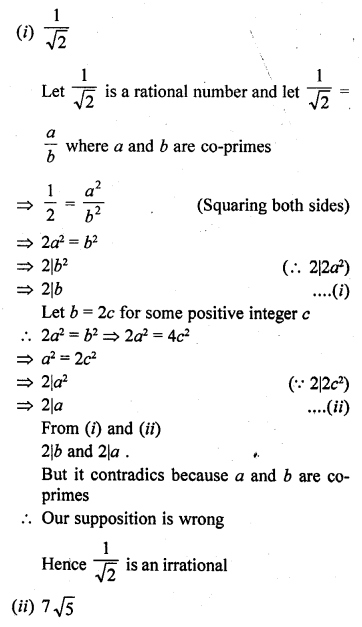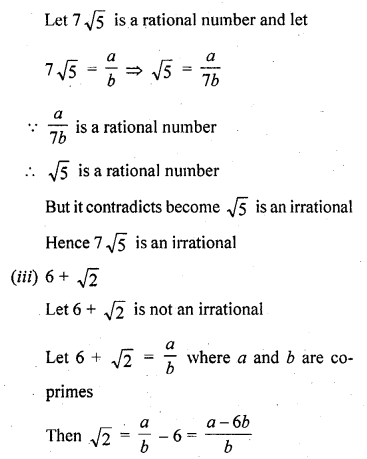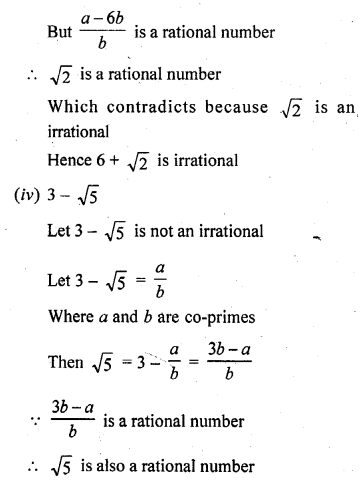But itcontradics that because √5 is irrational
3 – √5 is irrational

Question 2 :
Prove that following numbers are irrationals :
(i) 2/√7
(ii) 3/2√5
(iii) 4 + √2
(iv) 5 √2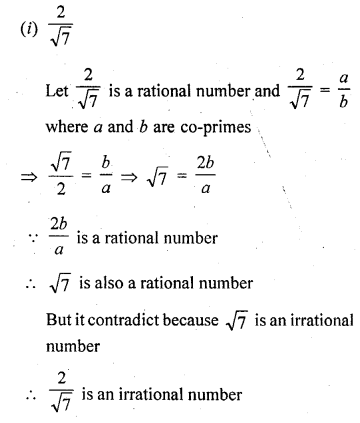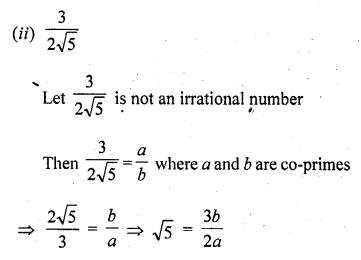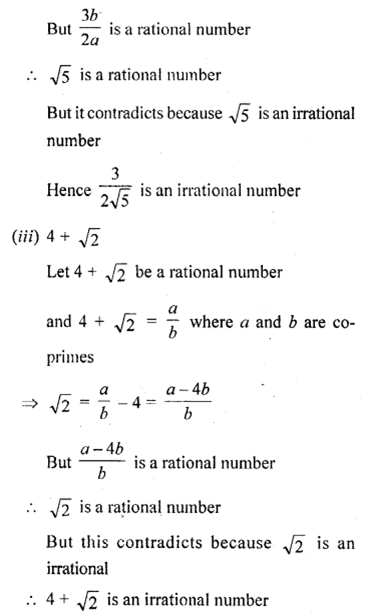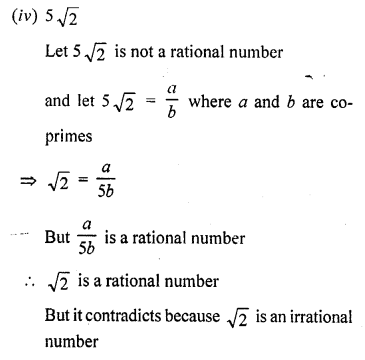5 √2 is an irrational number

Question 3 : Show that 2 – √3 is an irrational number. [C.B.S.E. 2008]

Let 2 – √3 is not an irrational number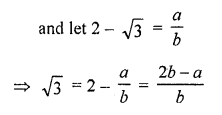√3 is a rational number
But it contradicts because √3 is an irrational number
2 – √3 is an irrational number
Hence proved.

Question 4 : Show that 3 + √2 is an irrational number.

Answer 4 : Let 3 + √2 is a rational number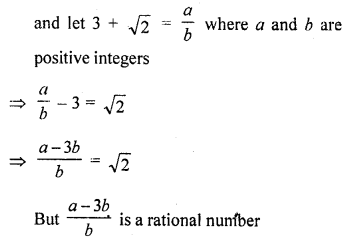and √2 is irrational
But our suppositon is wrong
3 + √2 is an irrational number

Question 5 : Prove that 4 – 5√2 is an irrational number. [CBSE 2010]

Let 4 – 5 √2 is not are irrational number
and let 4 – 5 √2 is a rational number
and 4 – 5 √2 = a/b where a and b are positive prime integers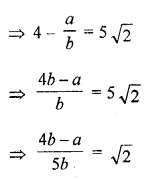√2 is a rational number
But √2 is an irrational number
Our supposition is wrong
4 – 5 √2 is an irrational number

Question 6 : Show that 5 – 2 √3 is an irrational number.

Let 5 – 2 √3 is a rational number
Let 5 – 2 √3 = ab where a and b are positive integers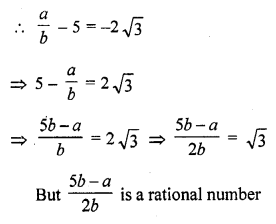and √3 is a rational number
Our supposition is wrong
5 – 2 √3 is a rational number

Question 7 : Prove that 2 √3 – 1 is an irrational number. [CBSE 2010]

Let 2 √3 – 1 is not an irrational number
and let 2 √3 – 1 a ration number
and then 2 √3 – 1 = a/b where a, b positive prime integers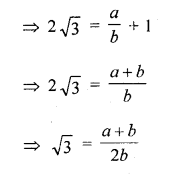√3 is a rational number
But √3 is an irrational number
Our supposition is wrong
2 √3 – 1 is an irrational number

Question 8 : Prove that 2 – 3 √5 is an irrational number. [CBSE 2010]

Let 2 – 3 √5 is not an irrational number and let 2 – 3 √5 is a rational number
Let 2 – 3 √5 = a/b where a and b are positive prime integers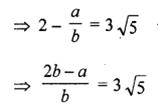⟹2b−a3b=√5
√5 is a rational
But √5 is an irrational number
Our supposition is wrong
2 – 3 √5 is an irrational

Question 9 : Prove that √5 + √3 is irrational.

Let √5 + √3 is a rational number
and let √5 + √3 = a/b where a and b are co-primes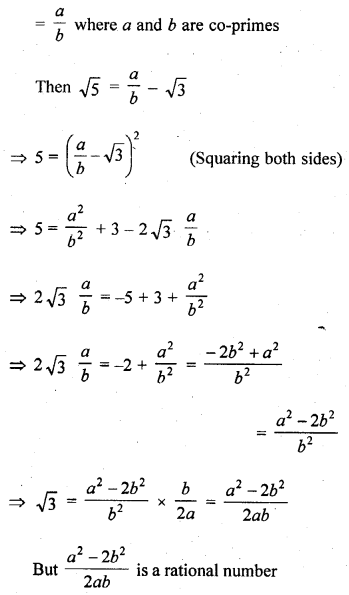√3 is a rational number
But it contradics as √3 is irrational number
√5 + √3 is irrational

Question 10 : Prove that √2 + √3 is an irrational number.

Let us suppose that √2 + √3 is rational.
Let √2 + √3 = a, where a is rational.
Therefore, √2 = a – √3
Squaring on both sides, we get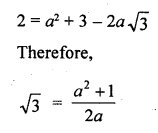which is a contradiction as the right hand side is a rational number while √3 is irrational.
Hence, √2 + √3 is irrational.

Todays Deals### RD Chapter 1- Real Numbers Ex-1.5 Contributorskrishan

Name:
Email:

# Latest News# 9000 interview questions in different categories